Next: Metropolis algorithm Up: Monte Carlo methods Previous: Rejection sampling

### Importance sampling

In this method, too, we start from an easy' function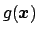, which we hope' will approximate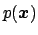of interest, of which in fact we know only its un-normalized expression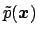. However, there is no requirement about howapproximates(but the goodness of approximation will have an impact on the efficacy of the method), apart from the condition that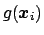must be positive wherever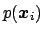is positive .

The functioncan be used in the calculation of, if we notice thatcan be rewritten as follows: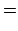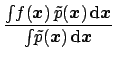(112)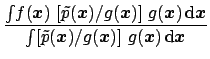(113)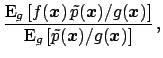(114)

where the the symbol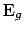is a reminder that the expectation is calculated over the distribution. Finally, the strategy can be implemented in the Monte Carlo using Eq. (111) for the two expectations: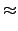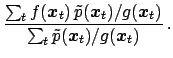(115)

From the same sample it is also possible to evaluate the normalization constant, given by the denominator of Eq. (113), i.e.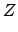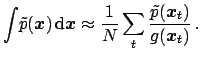(116)

The computation of this quantity is particularly important when we are dealing with model comparison and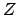has the meaning of evidence' (Sect. 7).

It easily to see that the method works well ifoverlaps well with. Thus, a proper choice ofcan be made by studying where the probability mass ofis concentrated (for example finding the mode of the distribution in a numerical way). Often a Gaussian function is used for, with parameters chosen to approximatein the proximity of the mode, as described in Sect. 5.10. In other cases, other functions can be used which have more pronounced tails, like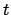-Student or Cauchy distributions. Special techniques, into which we cannot enter here, allowindependent random numbers to be generated and, subsequently, by proper rotations, turned into other numbers which have a correlation matrix equal to that of the multi-dimensional Gaussian which approximates.

Note, finally, that, contrary to the rejection sampling, importance sampling is not suitable for generate samples of unweighted events', such as those routinely used in the planning and the analysis of many kind experiments, especially particle physics experiments.Next: Metropolis algorithm Up: Monte Carlo methods Previous: Rejection sampling
Giulio D'Agostini 2003-05-13